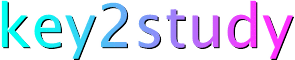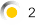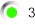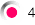Maths: On-line calculator

You can use our simple on-line calculator in just the same way as you would use an ordinary calculator. We have also included a few examples for you to try!

 Example 1Evaluate the expression:(7.5+2.7)/(1.9-0.3) Hint: Enter the entire expression and then click on the '=' key Example 2Find the square root of:79561 Hint: Enter the number and then click on the 'sqrt' key Example 3Solve the equation:x = sin(1.2) Hint: Enter the angle, 1.2 (expressed in radians), and then click on the 'sin' key Example 4Find the value of:e3.5 Hint: Enter 3.5 and then click on the 'exp' key

Home